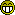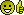###Author Topic: Project Euler  (Read 665 times)

#### Greenbank##### Project Euler
« on: January 01, 2021, 05:26:31 pm »
Similar to AdventOfCode there is the https://projecteuler.net/ set of problems. A new one is released every week (although week + 3 hours so that it doesn't favour a single timezone).

I've just joined up and done a bunch of the easy problems. They soon start to get a bit harder though.

My plan is to catch up with the backlog and do all of them, but this is a long term goal as there are 741 problems (as of today) and they do get quite tricky.

They ask for people not to publish the solutions of anything beyond problem 100.

Also they suggest that every problem should be solvable in under a minute of CPU time. If it's taking longer than that then you should be looking at a more efficient algorithm. I've got one that took 7 minutes as I used the naive solution and so I'll go back and implement it properly at a later date (I've got a running TODO list of ones to go back and look at).

Add me as a friend: 1774463_o9tD6r2BkAYDnoLfz3Sn79sfrUclAOxh and we can have a YACF league table.
"Yes please" said Squirrel "biscuits are our favourite things."

#### Ben T##### Re: Project Euler
« Reply #1 on: January 02, 2021, 09:19:27 am »
I've done quite a few, I think the hardest one I've done is
https://projecteuler.net/problem=579
It was very hard. I think my code takes a lot longer than a minute, but not like days. Something in the region of an hour IIRC. But the point is it's not building up memory and crashing.
Not sure I could do it again. It requires a bit of maths theory but aside from that it is about looking at combinations once and once only, in order to not have to keep track of them all in memory.

Some others that are hard, but must be doable cos I've done them

https://projecteuler.net/problem=615
https://projecteuler.net/problem=618
https://projecteuler.net/problem=632
https://projecteuler.net/problem=652
https://projecteuler.net/problem=643

Let me know any you manage to do and I'll have a go. Some are just a bit too theoretical, my only complaint is they're more a test of maths than of programming, but they're good.

Added you, my key is 1076128_GNwjy8pJQE6yQge9Iy2sowUSHYfRGJ7Z
It is what it is. It's not what it's not, so it must be what it is.

#### Greenbank##### Re: Project Euler
« Reply #2 on: January 02, 2021, 12:00:15 pm »
Let me know any you manage to do and I'll have a go. Some are just a bit too theoretical, my only complaint is they're more a test of maths than of programming, but they're good.

Good to know. I don't fear the theoretical ones, in fact I kind of prefer them. It's one benefit of having a Maths degree and a Comp Sci degree.
"Yes please" said Squirrel "biscuits are our favourite things."

#### Lightning Phil##### Re: Project Euler
« Reply #3 on: January 02, 2021, 12:58:28 pm »
First one is indeed easy. Three additions and two divisions to get the answer. Might join this at some point. I’m also a maths graduate#### freeflow##### Re: Project Euler
« Reply #4 on: January 02, 2021, 10:22:06 pm »
Interesting

1-10 completed

my friend key is

1774618_CwG4VPhUG2pCIv66g4t37hF8pjGDzK9v

#### Greenbank##### Re: Project Euler
« Reply #5 on: January 03, 2021, 07:58:56 pm »
I've done 35 of the top 50 "easiest" (as in solved by most people), only because I ordered them by "solved by number" and didn't switch it back.

I plan on finishing off the top 50 "easiest" and the remaining ones in the first 50, then I'll pick and choose my way through with a mixture of tough ones and easier ones.

Hoping to try and keep up a pace of ~50 a week.
"Yes please" said Squirrel "biscuits are our favourite things."

#### Ben T##### Re: Project Euler
« Reply #6 on: January 03, 2021, 09:02:24 pm »
Ok here's a question I wonder if any maths boffins can help me with please?
It's to do with set theory/combinatorics.
I think this could come in handy for multiple PE problems.

How do I find out the total number of sets containing up to n in each, containing each of k distinct objects at least once (where k <= n) ?
So for instance, if n=5, k=3
You could have
{1 2 3}
{3 2 1}
{1 1 2 3}
{1 1 1 2 3}
{1 1 1 3 2}
{1 1 2 2 3}
{1 1 3 3 2}
{1 2 2 2 3}
etc
but you couldn't have
{1 1 1 2} (doesn't contain 3)
{3 3 3 3 3} (doesn't contain 1 or 2)
etc.
and the order matters, so {1 2 3} != {3 2 1}

I know it's something like
f(n,k) = k^n -  f(n,k, k-1) - f(n,k, k-2) - ... - f(n,k,1)

where f(n,k,m) is the number of sets of n from k where the total number of distinct elements is m.
(so therefore f(n,k) is always equal to f(n,k,k) )
and f(n,n) is always just n factorial, because it's the number of permutations of the distinct set of objects.

because k^n is the total permutations of n sets from 1..k, but then you have to discount all the ones where there are less than k distinct elements, i.e. not all the numbers are represented.

but what's f(n,k,m) ?

I've tried an implementation that says

f(n,k)
{
if(n==k) return n!;

res = k^n;
for(m = k-1; m > 0; m--)
{
res -= f(n,k,m);
}
}

f(n,k,m)
{
if(m == 1) return k;
return k * f(n,k)
}

and that works for any k when n <= 5
and for f(6,1), f(6,2), f(6,3)

but it fails for f(6,4).

It returns f(6,4,2) = 2160 when it should be 372.

any ideas?
It is what it is. It's not what it's not, so it must be what it is.

#### Ben T##### Re: Project Euler
« Reply #7 on: January 04, 2021, 10:44:52 am »
ooh yes, a breakthrough - figured out that f(n,k) == k . [ f(n-1, k) + f(n-1, k-1) ]
so f(6,4) = 4. [ f(5,4) + f(5,3) ]
= 6 x (240+150) = 6x390 = 1560

should hopefully help solving one of the harder ones

writing out numbers in a grid often helps you to see patternsIt is what it is. It's not what it's not, so it must be what it is.

#### Ben T##### Re: Project Euler
« Reply #8 on: January 04, 2021, 03:10:46 pm »
anybody any hints on https://projecteuler.net/problem=734
?

I can get the answer from the total number of distinct sets of ascending primes, to the answer, using the formula above
so for their example T(5,2)
I can get from
{2}
{2,3}
{3}
{5}